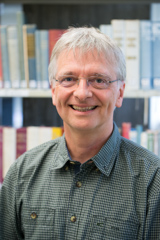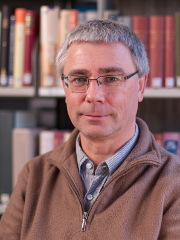# Teaching

The ITLR teaches thermodynamics, heat transfer, heat radiation and droplet dynamics for Bachelor and Master students in aerospace engineering.

### The teaching at the ITLR

The ITLR offers a wide range of courses. The exams and consultation hours are updated regularly.

Our employees regularly propose job offers in science or teaching. Furthermore there are offers for Bachelor and Master theses.

### Topics of the courses

The lectures given at the ITLR are mainly concerned with thermodynamics. Thermodynamics consists of the Greek words thermós (heat) and dýnamis (dynamic). It is the science of energy conversion (e.g. from thermal to mechanical energy), energy flows and heat transfer.

These processes are described with macroscopic system variables (such as temperature, pressure, density, heat, volume). Thus a continuum is considered and no attempt is made to describe the dynamics of individual particles (such as atoms or molecules). Theoretically, the processes should therefore run so slowly that all quantities have the possibility to regain a state of equilibrium at any time. In reality, however, it can be seen that the laws of thermodynamics still provide very good approximations even for processes that strongly change over time (such as flow or combustion in a gas turbine or in a rocket engine).

Thermodynamics is therefore used in numerous energy-generating systems. These include steam power plants, fuel cells, thermoelectric coolers (e.g. refrigerators), turbines or piston and rocket engines.

Thermodynamic investigations are gaining importance as they are fundamental for the analysis, development and design of energy generating systems. Important engineering objectives such as increased efficiency, improved design, optimal operating conditions, reduced environmental impact and alternative methods of power generation are made possible by the application of the fundamentals of thermodynamics.

### Lectures in B.Sc.

The lectures Thermodynamik I&II present the fundamentals of classical thermodynamics from the point of view of continuum theory. In addition to the three main theorems of thermodynamics, which form the core of the lecture, the following topics are covered in the B.Sc. course of studies:

Concept of a function of state, equations of state, internal energy, enthalpy, entropy, free energy, free enthalpy, equilibrium conditions, Maxwell relations, multiphase systems, Clausius-Clapeyron equation and the Gibbsian phase rule.

Furthermore, the lectures in the second part detail important applications of the laws of thermodynamics. It deals with the cyclic processes of the working and thermal engines with special consideration on the aerospace industry.

The thermodynamic equilibrium conditions are applied to the chemical equilibrium and to the phase equilibrium at different pressures : chemical potential, law of mass action, principle of least constraint, theorem of Hess, heterogeneous reactions, Kirchhoff's equation and catalysis are treated here. Thermodynamics is also applied to simple flow processes: weak disturbances of the state variables, the speed of sound and changes of state over the compression impact are considered.

### Lectures in M.Sc.

The compulsory lecture Analytical and Numerical Methods deals with the basics of mathematical modelling and methods of applied mathematics. The goal here is the calculation of solutions for partial differential equations.

Analytical methods include dimensional analysis, perturbation calculation, mathematical modelling with differential equations as well as solutions for simple partial differential equations, Fourier series and transformations, separation approaches and conservation equations.

Based on the fundamentals of numerical mathematics, the construction principles of numerical methods are explained. The analytical and numerical tools are used for the determination of solutions and approximate solutions. These are stationary heat conduction problems, transient diffusion, heat conduction and wave propagation. Finite volume, finite element and difference methods are derived and applied.

In addition to the compulsory lecture, the ITLR also offers a number of specialization modules. Among them are the kinetic gas theory, heat transfer intensification, selected problems of heat transfer, basics of quantum mechanics and many more.

### Contact Persons### Bernhard Weigand

Prof. Dr.-Ing. habil.

DirectorProf. Dr.-Ing.

Deputy director